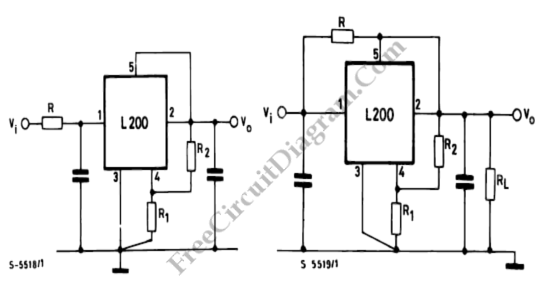# Reducing L200 Power Dissipation using Series ResistorIt’s good to reduce the power dissipated by the device. Using resistor connected in series to the input (the left figure) is a simple and economic method to reduce the device input-output differential voltage. Here’s the formula for calculating R:

R= [Vi min- (Vo+Vdrop)]/Io

Vdrop = minimum differential voltage between the input and the output of the device at current Io

Vin min = minimum voltage

Vo = Output voltage

Io = output currentResistor R can be connected between pins 1 and 2 of the IC instead of in series with the input if the load is constant (the right figure). So, part of the load current flows through the device and part through the resistor. This configuration is available when the minimum current by the load is:

Io min = Vdrop/R

[Source: SGS-Thomson Microelectronics Application Note]